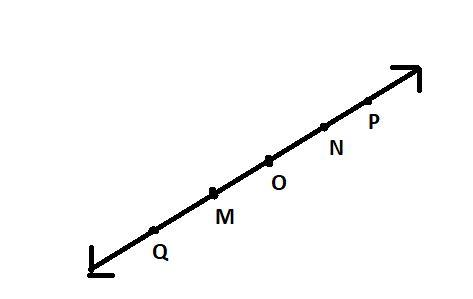Courses
Courses for Kids
Free study material
Free LIVE classes
MoreLIVE
Join Vedantu’s FREE Mastercalss

# Consider the following figure of line$\overline {MN}$. Say whether following statement is true or false in context of the given figureRay $\overrightarrow {OP}$is different from ray$\overrightarrow {QP}$.$A.$True$B.$FalseVerified
Here, ray$\overrightarrow {OP}$and $\overrightarrow {QP}$ have same direction but different initial point so they are different